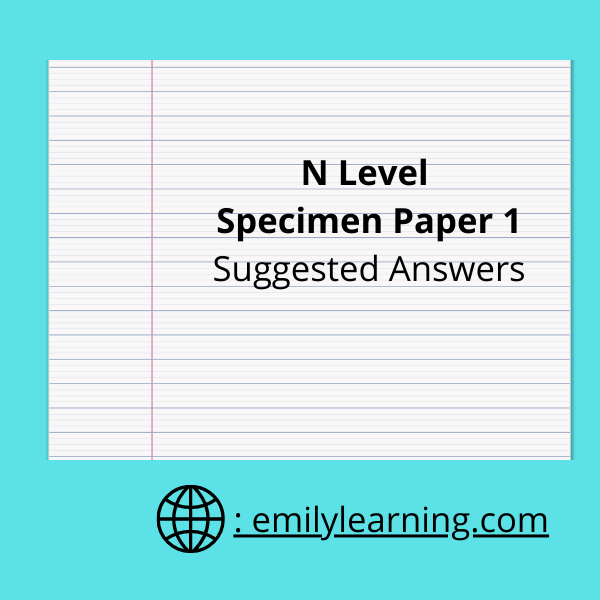Click on question below to go directly to the question you want below:

Question 1

Question 2

Question 3

Question 4

Question 5

Question 6

Question 7

Question 8

Question 9

Question 10

Question 11

Question 12

## N Level Specimen Paper 1 Question 1 On Surds

The first question is on surds, and students are required to rationalize the denominator.

## N Level Specimen Paper 1 Question 2 On Quadratic Functions

In this question, students apply the concepts of discriminant and solving of quadratic inequalities.

## N Level Specimen Paper 1 Question 3 on Trigonometry

Trigonometry covers many different concepts. In this question, curve sketching is tested.

## N Level Specimen Paper 1 Question 4 on Cubic Equations

In this question, students are required to factorize a cubic expression.

## N Level Specimen Paper 1 Question 5 on Differentiation

In this question, students are tested on application of differentiation, applying it to stationary points.

## N Level Specimen Paper 1 Question 6 on Trigonometry

Another trigonometry question in paper 1. In part a, students are tested on using trigonometric identity (found in the formula sheet) and also on special angles. In part b, students are expected to solve trigonometric equations.

## N Level Specimen Paper 1 Question 7 on surds

In this question, you will be solving equations involving x. However, as you are required to give answers in exact form, students will need to simplify surd expressions.

## N Level Specimen Paper 1 Question 8 on Trigonometry

For this question students are given a trigonometric curve, and asked to determine its equation.

## N Level Specimen Paper 1 Question 9 on Differentiation and Integration

In the first part of the question, students are asked to differentiate an expression. In part b, students are asked to apply the concept of integration is the reverse of differentiation to integrate the expression.

## N Level Specimen Paper 1 Question 10 on simultaneous equations

This question involves setting up 2 equations involving r and h and solving for them.

## N Level Specimen Paper 1 Question 11 on coordinate geometry

In this question, students are tested on concepts learned in the chapter on coordinate geometry such as finding gradients, equation of lines and area of polygons.

## N Level Specimen Paper 1 Question 12 on Integration

In this question, students are required to find an equation y by integration.

## Learn On-demand – A- Math Courses

If you want to learn a particular topic in detail, check out our on-demand O Level A Math courses here.

Suggested Answers for other A Math Papers: